# 銀河考古学总结

## 架起认知的桥梁

Posted by mingjie on October 26, 2018

べき指数 幂指数

ベータ崩壊 β衰变

## 1 序章

### 银河系的构造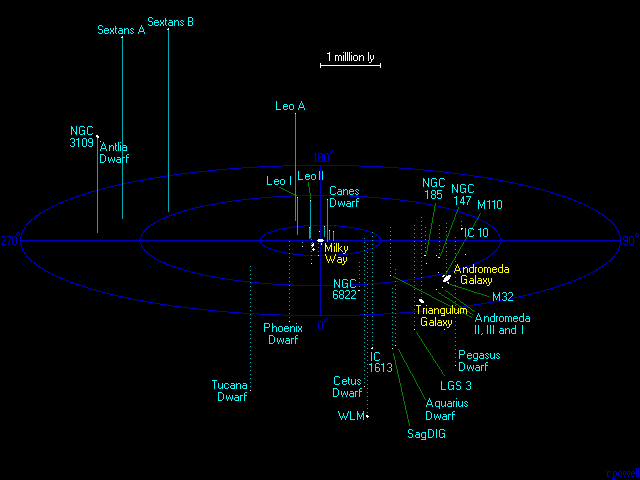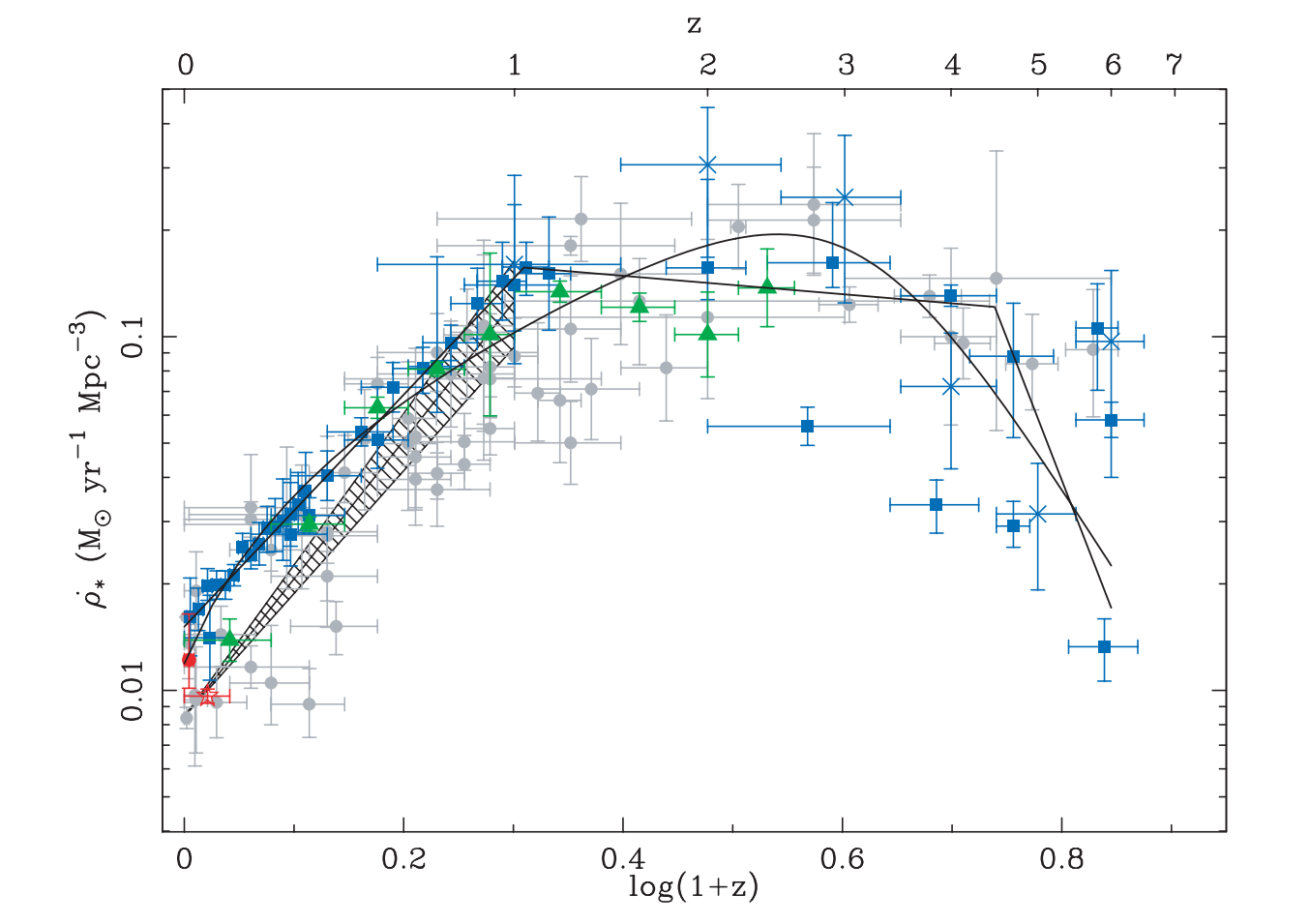恒星形成率密度与红移的关系；HB06

### 什么是银河考古学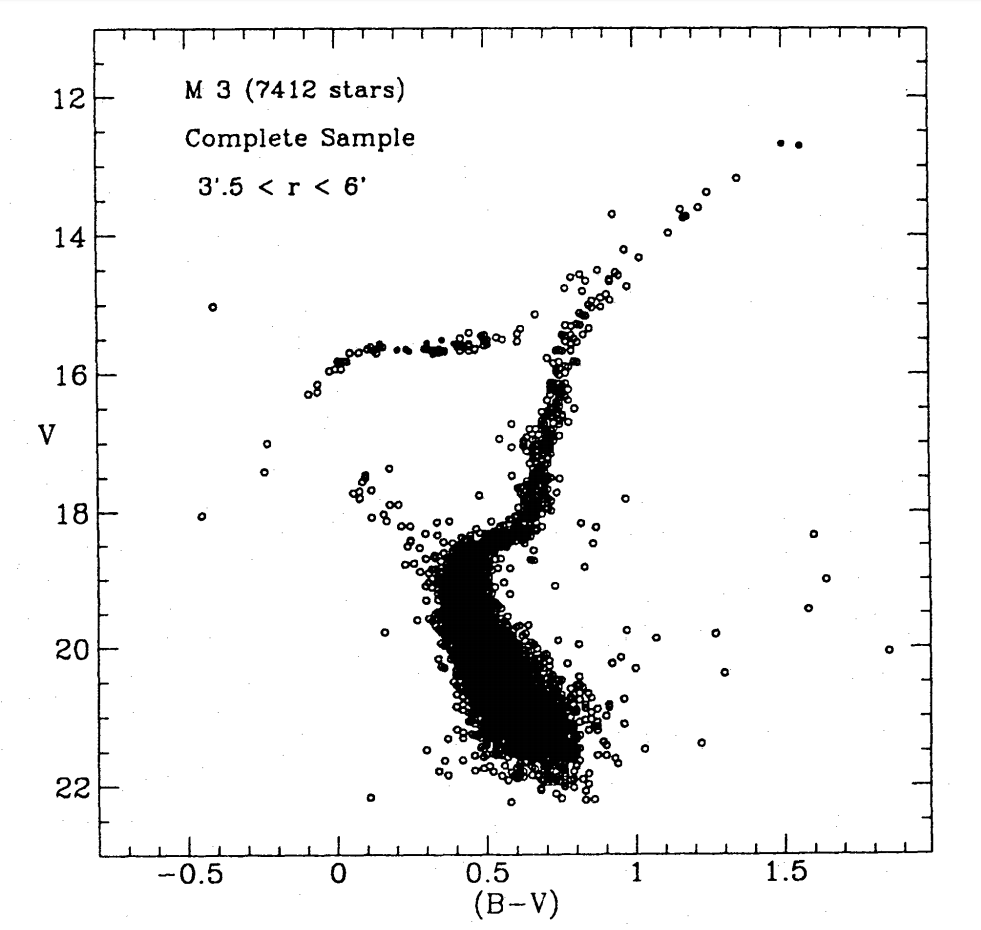Buonanno+1994

## 2 星系运动学与银河系动力学

### 恒星的轨道运动

#### 球对称引力势下的轨道

##### 运动积分

1. 被引力场束缚的轨道的运动积分$$\ge 0$$
2. 当引力势的变化时标与系统的动力学时标相比很大的时候，$$J_i$$是绝热不变量
3. 一个轨道群在相空间$$(\boldsymbol{r},\boldsymbol{v})$$所占的体积与它们在运动积分空间$$J_i$$所占的体积成比例

### 恒星运动学和银河系的自转

#### 太阳的信息怎么测？

$U_\odot = -11.10 \mathrm{km/s}, V_\odot = 12.24 \mathrm{km/s}, W_\odot = 7.25 \mathrm{km/s}$ $R_0 = 8.3 \mathrm{kpc}, \Theta_0 = 239 \mathrm{km/s}$

### 银河系引力势模型

$\rho(R,z) = \rho_0 e^{-R/h}e^{-|z|/z_0}$

### 恒星的理论力学和动力学

#### 太阳邻域的恒星速度

• 富金属星（银盘）的速度集中在$$(U,V)=0$$附近
• 贫金属星的$$V$$速度为负，且弥散比较大
• 极贫金属星（银晕）的速度集中在$$-\Theta_0$$附近且弥散大

## 恒星分类与演化

### 颜色星等图(CMD)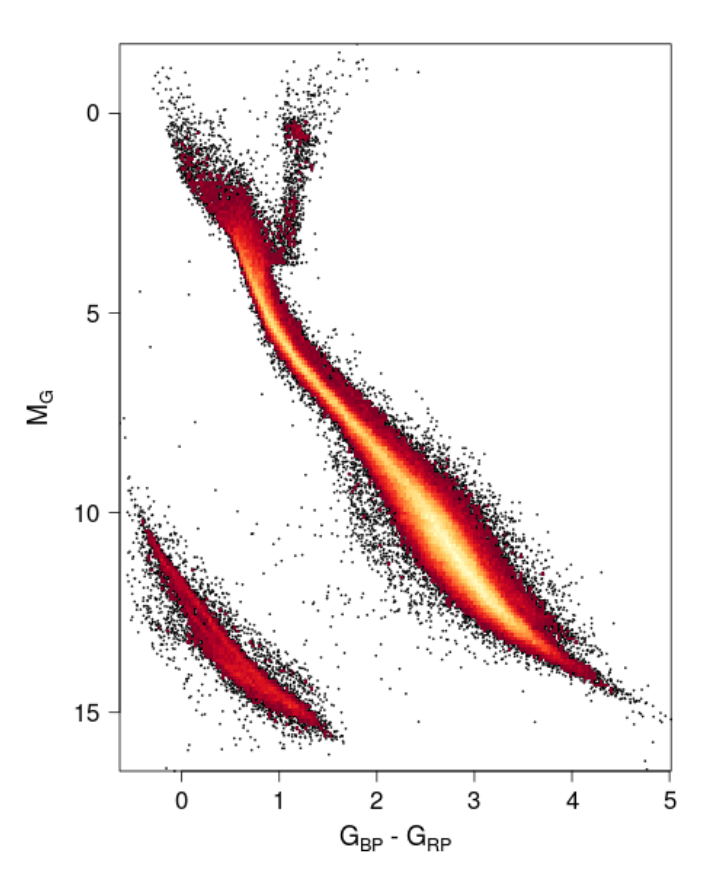太阳邻域(<100pc)内的恒星CMD；Gaia Collaboration+18, Fig. 6

### 恒星演化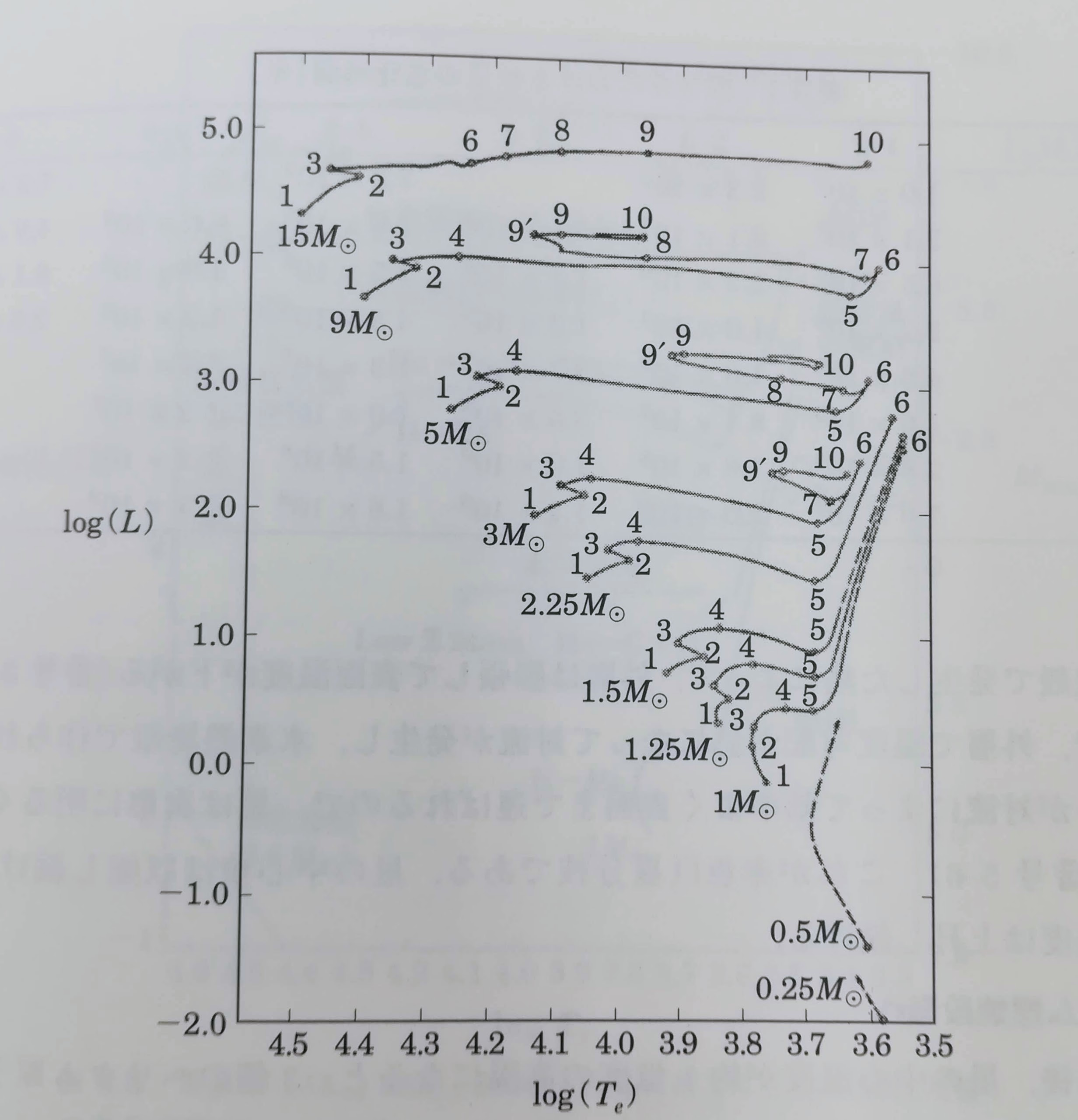### 元素的起源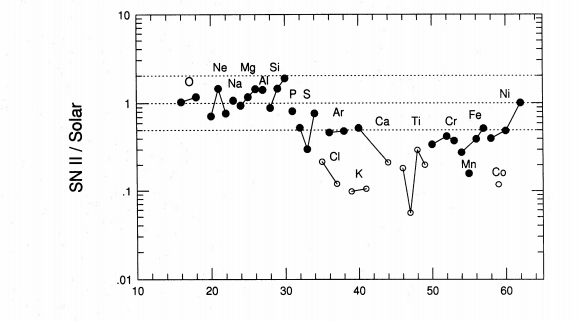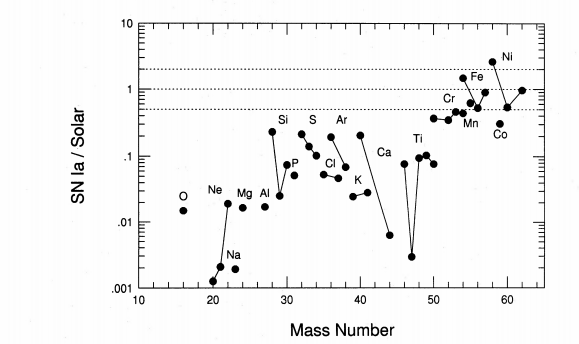Tsujimoto+95

#### 比铁更重的元素

Na、Al： 在恒星内部生成，与恒星初始质量、金属丰度有关；
Mg：在大质量恒星内部生成，与恒星初始质量有关，SN Ia基本不生成；

Ba、Pb：s过程；
Eu、Pt、Au、Th、U：r过程；

### 贫金属星和星族II

（190610更新）：现在基本上已经不怎么用星族这个简略的概念了，因为星族实际上不止三个，而且不同人对星族有不同的定义。

#### $$\alpha$$元素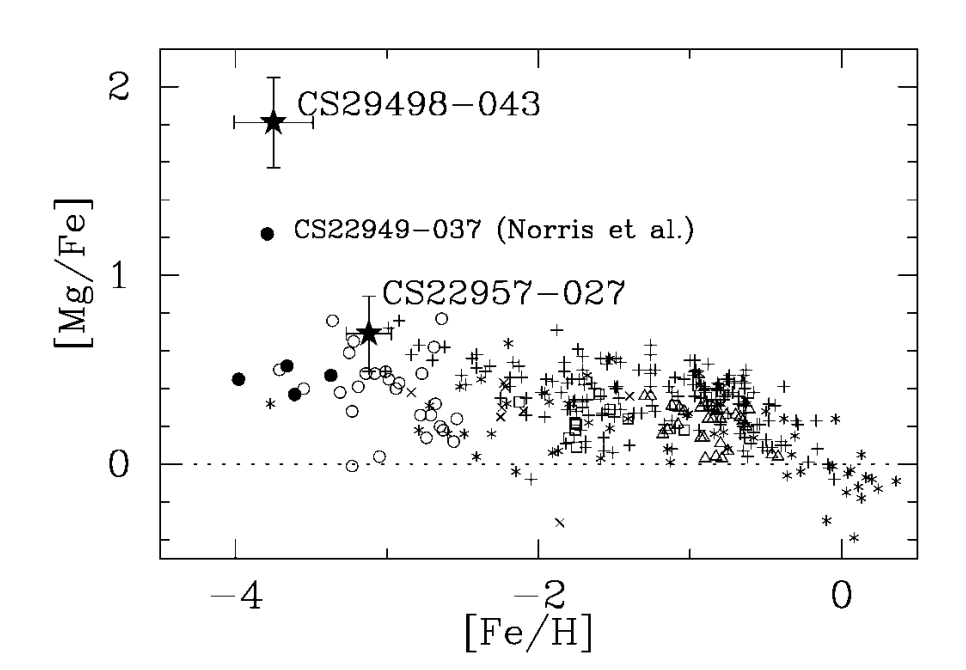Aoki+02, Fig. 2

• 金属丰度小于-1的恒星有正的[Mg/Fe]，说明是从早期的SN II中来的
• 金属丰度小于-2.5的恒星的[Mg/Fe]弥散大，这是由于早期SN II质量能量有弥散导致的，并没有经历多次的超新星爆发。
• 富金属端[Mg/Fe]接近0，说明新近的恒星遭受了SN Ia的污染

#### r、s过程元素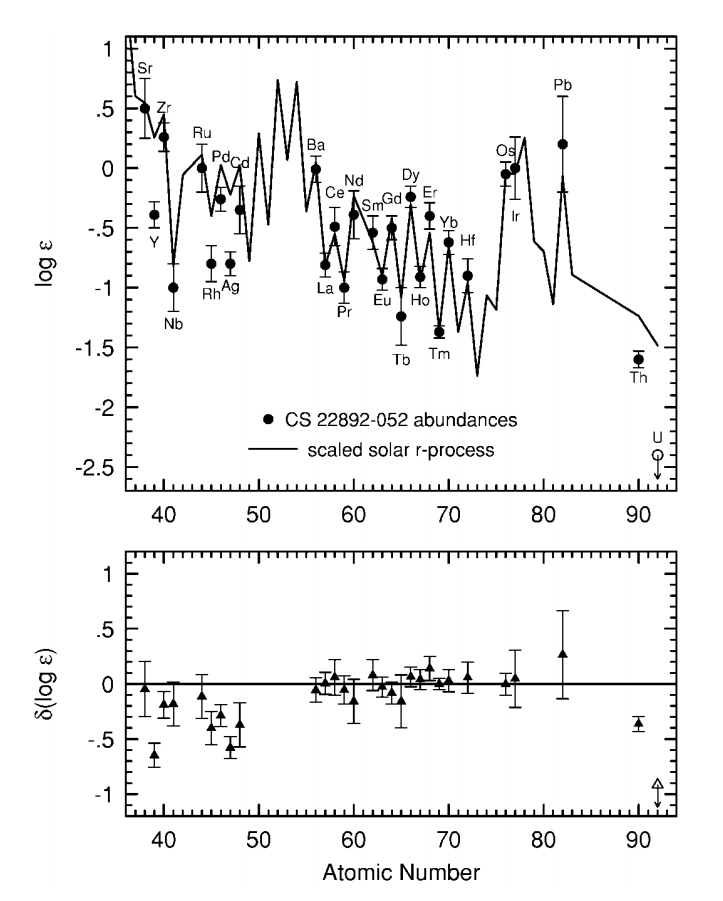贫金属星的r过程元素（点）与太阳的r过程元素（实线）比较；Sneden+00, Fig. 2

• r过程是普遍的，不论是在贫金属星还是富金属星上
• 这样的元素丰度应该来自于SN II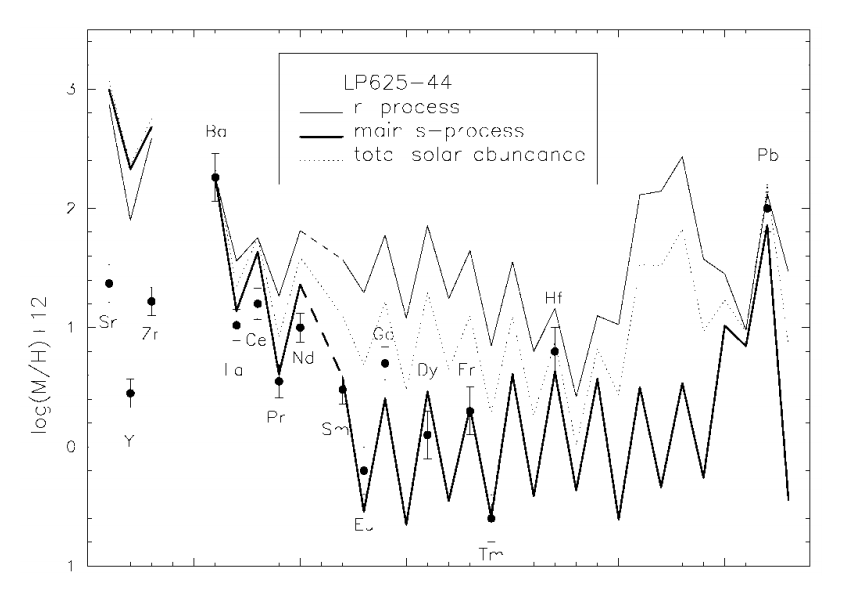贫金属星的s过程元素（点）与太阳的s过程元素（实线）比较；Aoki+00, Fig. 3

• s过程不是普遍的

## 银河系的化学演化

### 基本量

$\psi(t) \propto \rho_\mathrm{gas}^n$

$\phi(m) \propto m^{-\alpha}$

### 基本公式

SFR实际上是从星际介质到恒星中的质量的比例，那么定义$$e$$为从恒星到星际介质中的质量比例，$$F, E$$为星系星际介质从外界的流入的以及流出的比例，则恒星和星际介质的质量变化为：

$\frac{dM_\mathrm{s}}{dt} = \psi - e$ $\frac{dM_\mathrm{g}}{dt} = -\psi + e + F - E$

$$e$$可以表述为：

$e = \int_{m_\mathrm{t}}^\infty (m-m_\mathrm{rem})\psi(t-\tau_m)\phi(m)dm$

$$\tau_m, m_\mathrm{t}$$是质量为$$m$$的恒星寿命以及在时刻$$t$$下可以死亡的恒星的最小质量，$$m_\mathrm{rem}$$是恒星残骸的质量。$$e$$意思就是在时刻$$t$$下所有死亡恒星抛出的质量，所以这个积分有下限，同时还涉及到$$t-\tau_m$$这个时刻上产生的质量为$$m$$的恒星的比例$$\psi(t-\tau_m)\phi(m)$$。

$\frac{ZM_\mathrm{g}}{dt} = -Z\psi + e_Z +Z_F F - Z_E E$

$$Z_F, Z_E$$为流入流出的气体的金属丰度，$$e_Z$$可以表达为

$e_Z(t) = \int_{m_\mathrm{t}}^\infty [(m-m_\mathrm{rem})Z(t-\tau_m) + mp_\mathrm{zm}]\psi(t-\tau_m)\phi(m)dm$

$R = \int_{m_\mathrm{t}}^\infty (m-m_\mathrm{rem})\phi(m)dm$

$y = \frac{1}{1-R} \int_{m_\mathrm{t}}^\infty mp_\mathrm{zm}\phi(m)dm$

$$y=0$$意味着恒星对星际介质的金属丰度没有任何变化。

#### 瞬间循环近似(instantaneous recycling approximation)

$\frac{dM_\mathrm{s}}{dt} = (1-R)\psi$ $\frac{dM_\mathrm{g}}{dt} = -(1-R)\psi + F - E$ $\frac{ZM_\mathrm{g}}{dt} = -Z(1-R)\psi + y(1-R)e_Z +Z_F F - Z_E E \tag{3.25}$

### 简单模型

• 没有星际介质的流入流出；
• $$Z(0) = 0$$；
• IMF与时间无关
• 气体中的金属的空间分布是均匀的

$M_\mathrm{g}\frac{dZ}{dM_\mathrm{g}} = -y \Rightarrow Z = y \ln{\frac{M_\mathrm{g}(0)}{M_\mathrm{g}(t)}} = y \frac{1}{\ln{\mu}}$

$S = \frac{M_\mathrm{s}}{M_\mathrm{s1}} = \frac{1-\mu_1^{Z/Z_1}}{1-\mu_1}$

$\frac{dS}{d\mathrm{[Fe/H]}} = \frac{\ln{10}/y}{1-e^{-Z_1/y}} 10^{\mathrm{[Fe/H]}} e^{-10^{\mathrm{[Fe/H]}}/y}$

#### 有气体流入流出的银河系

##### 仅有流出

$E = c\psi(t)$

$Z = y \frac{1-R}{1-R+c} \frac{1}{\ln{\mu}}$

##### 仅有流入

$F = (1-R) \psi$

$Z = y(1-e^{-M_\mathrm{s}/M_\mathrm{g}})$

## 4 银河系的构造

### 核球

#### CMD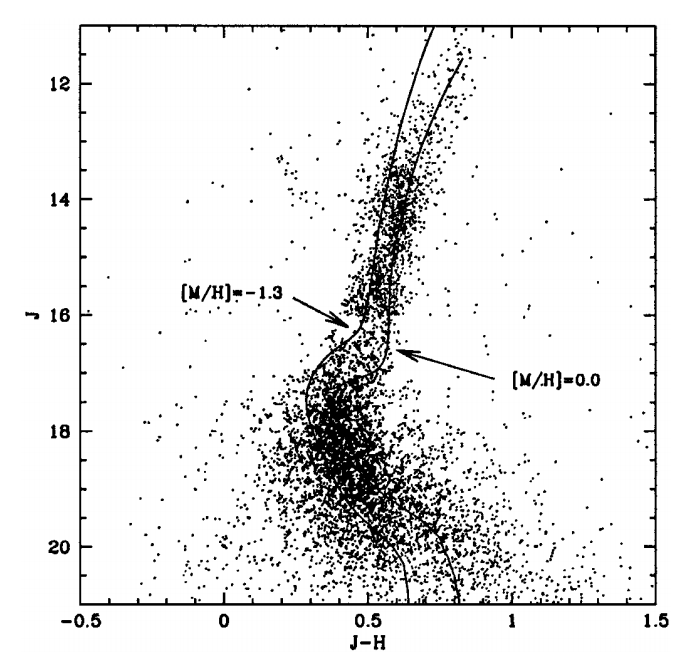Zoccali+03, Fig. 24

#### 金属丰度和元素丰度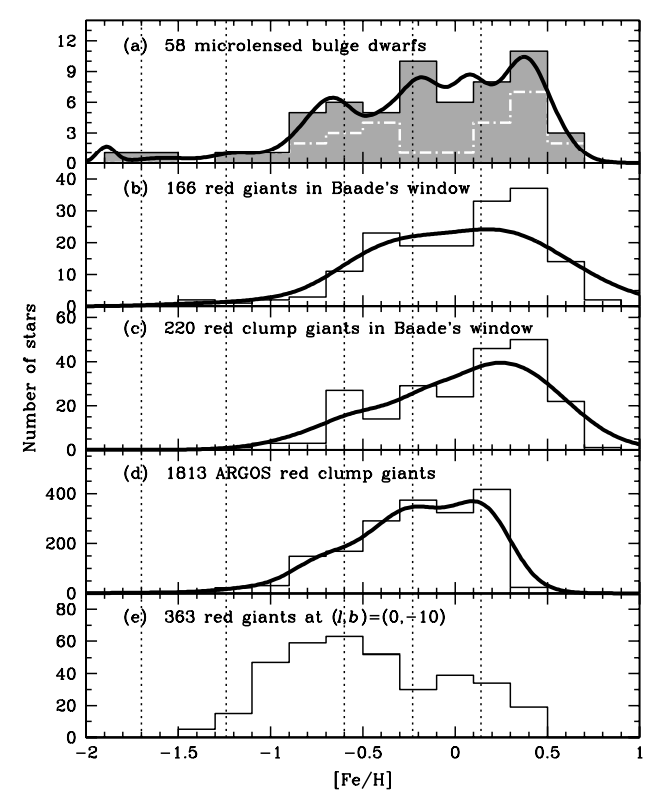Bensby+13, Fig. 14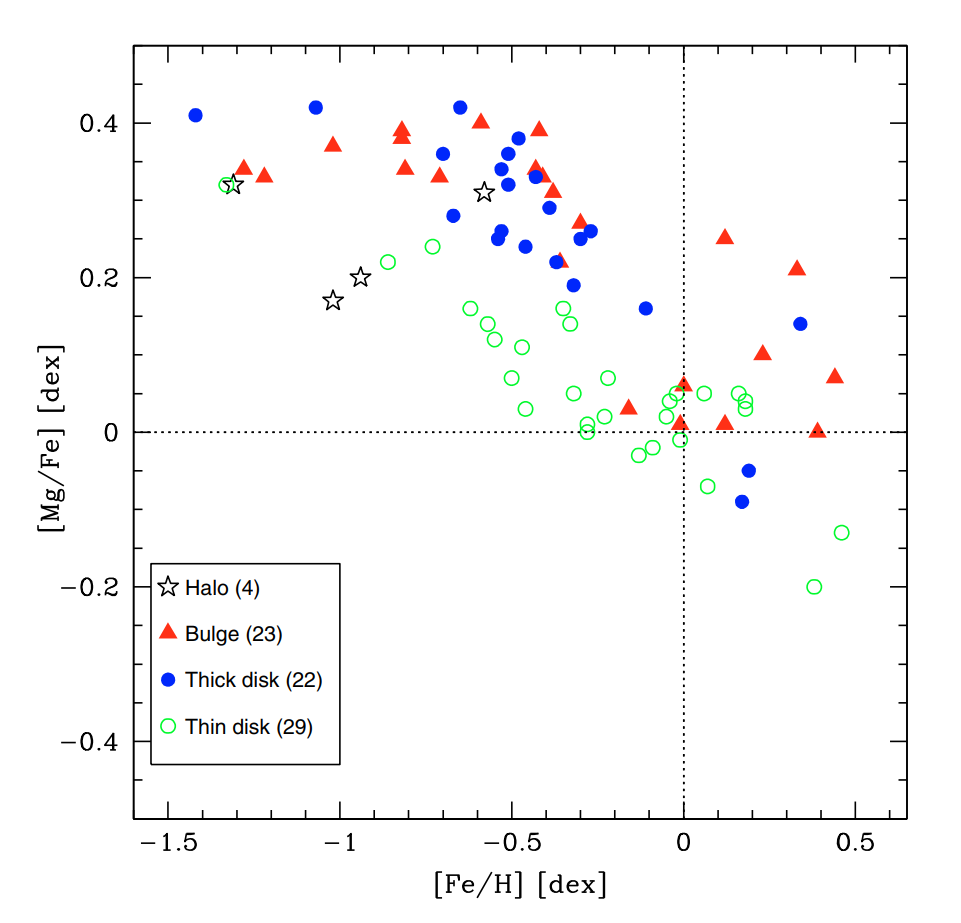Alves-Brito+10, Fig. 6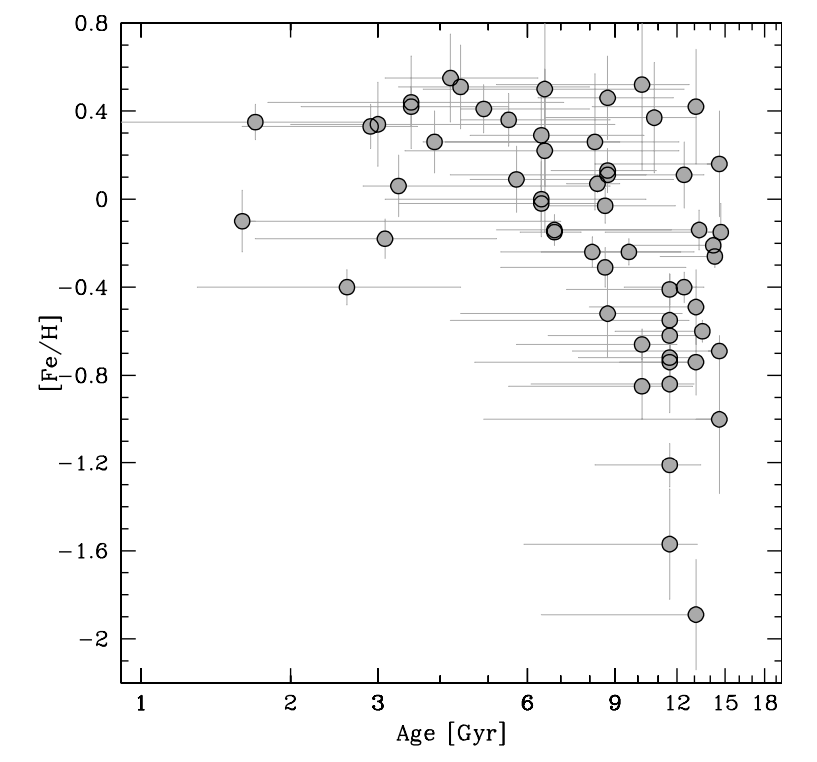Bensby+13, Fig. 15

#### 动力学构造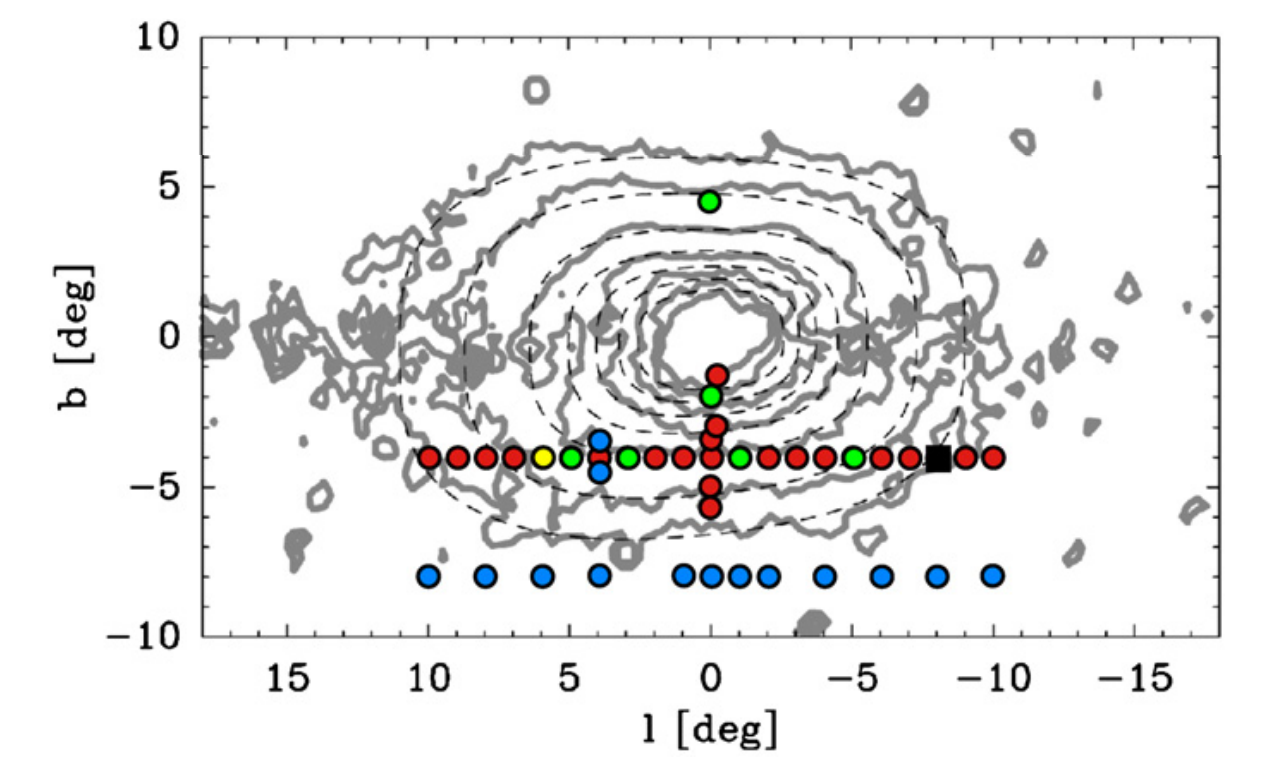Howard+09, Fig. 1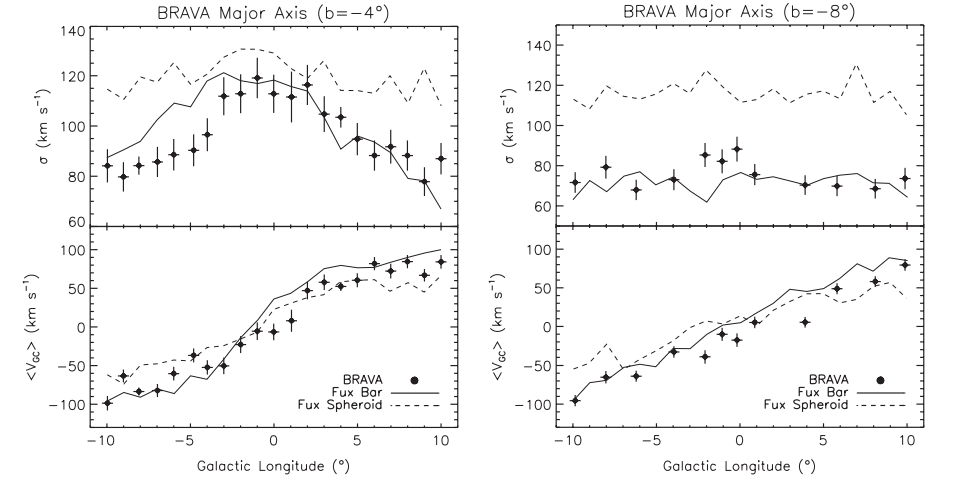Howard+09, Fig. 5

### 银盘

#### 厚盘的发现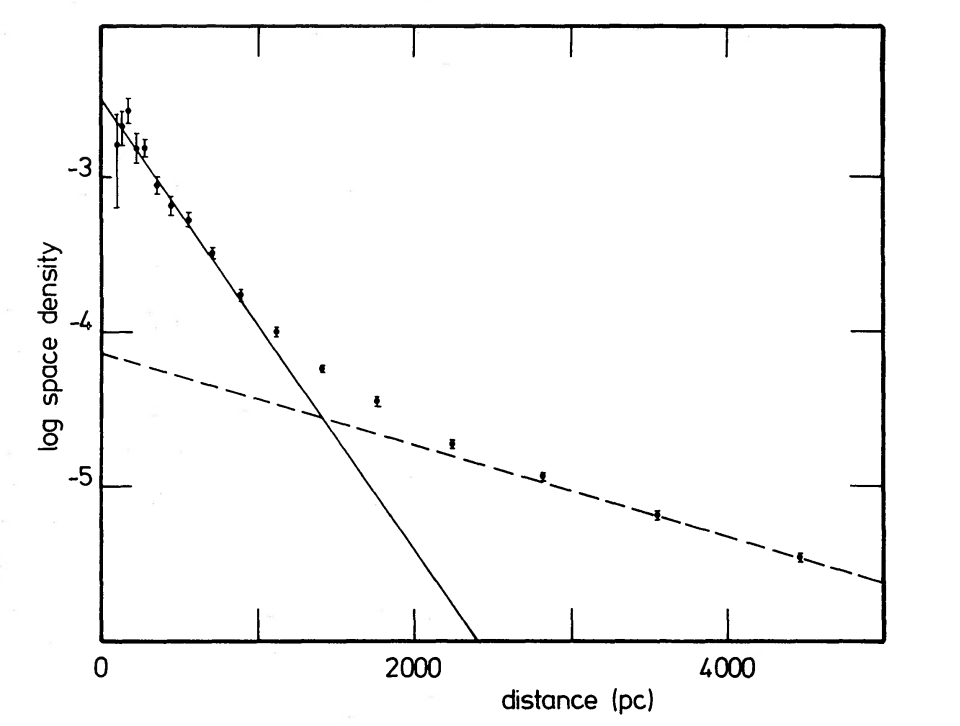#### 银盘的动力学构造

Edvardsson+93有一篇引用超多的论文讨论太阳邻域恒星的位置元素丰度年龄等等的关系。元素丰度与W速度的关系或许能说明一些情况：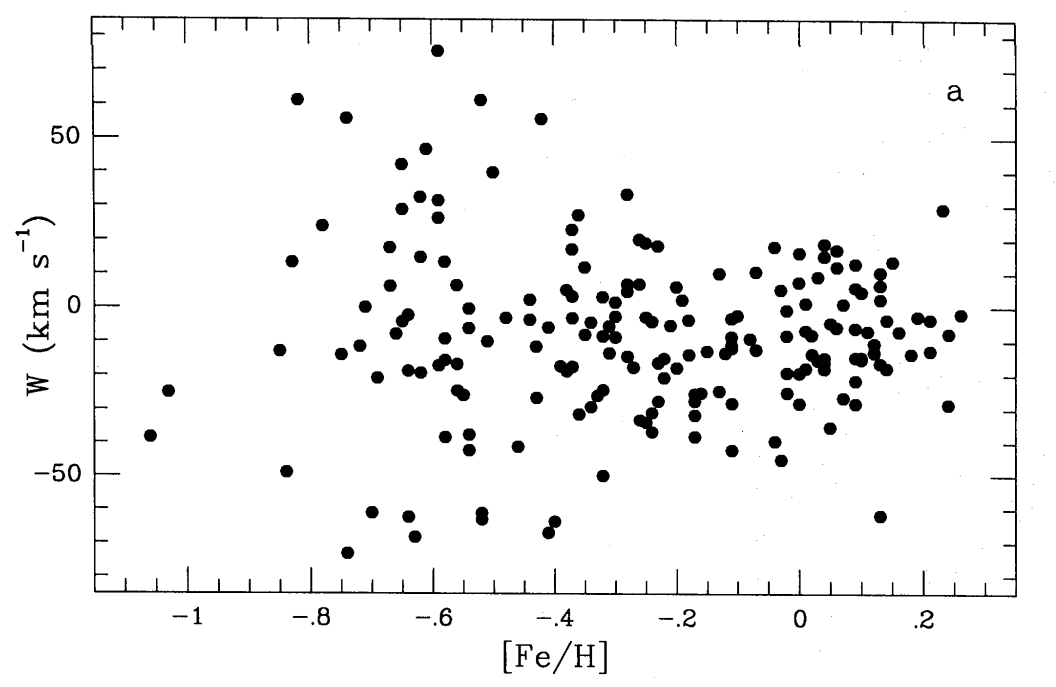#### 金属丰度以及化学元素组成

Edvardsson+93虽然得出了太阳邻域厚盘以及薄盘恒星的金属丰度，但是它们是混在一起的。Wyse & Gilmore 1995用太阳邻域样本和远处样本比较的方法将两者分离，并得出了银河系各成分的金属丰度分布：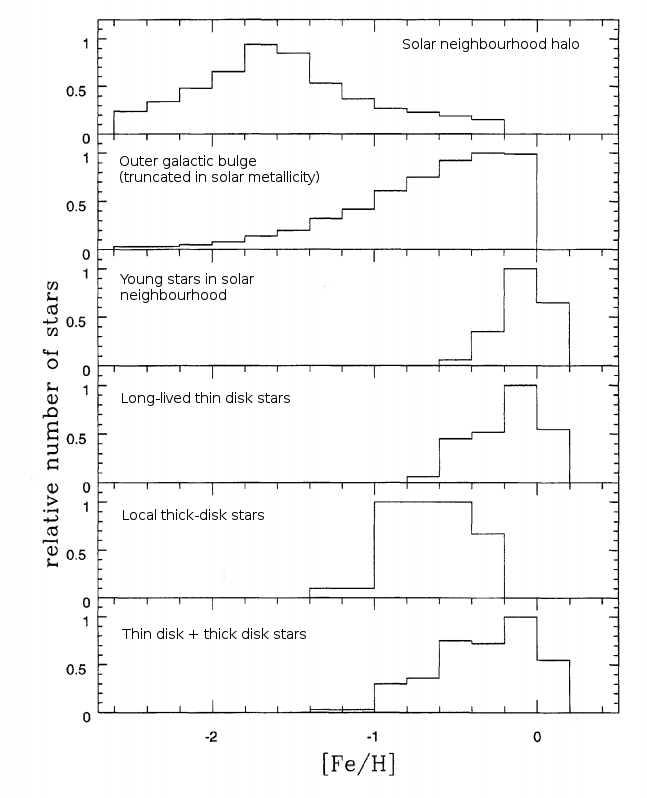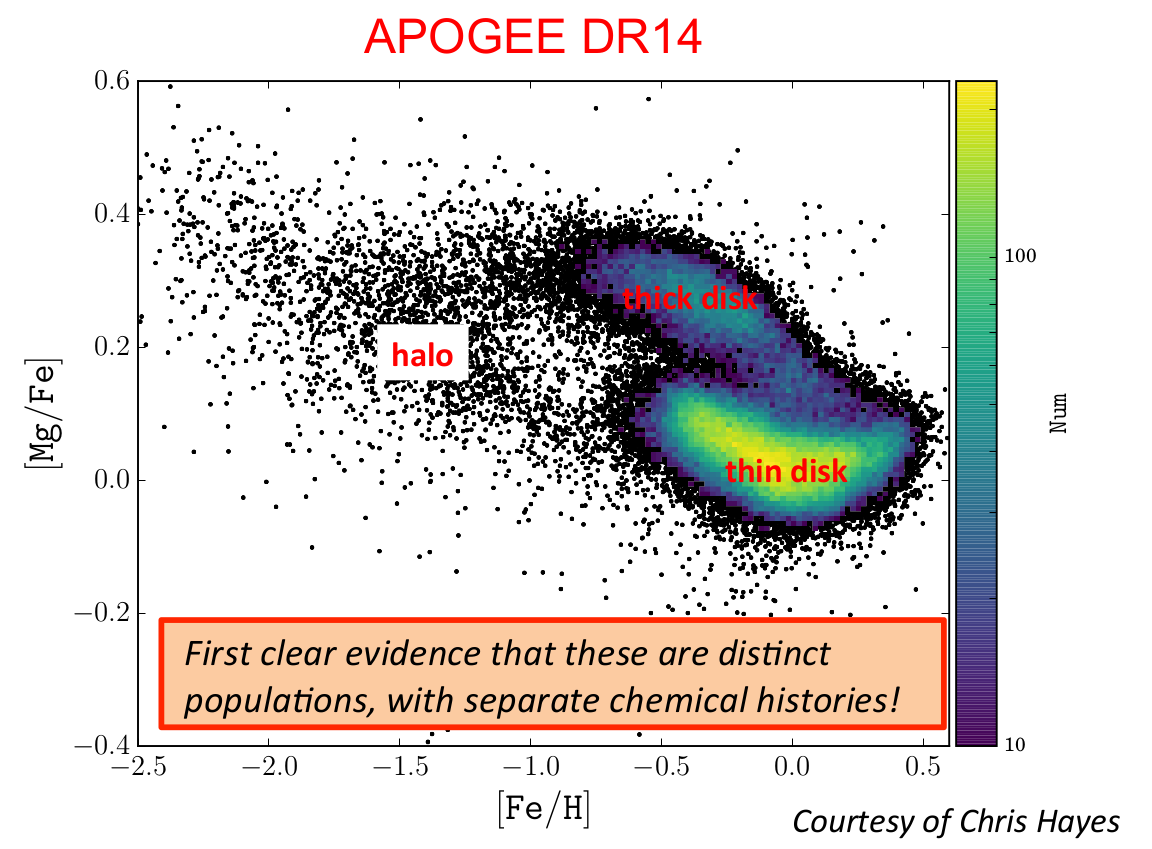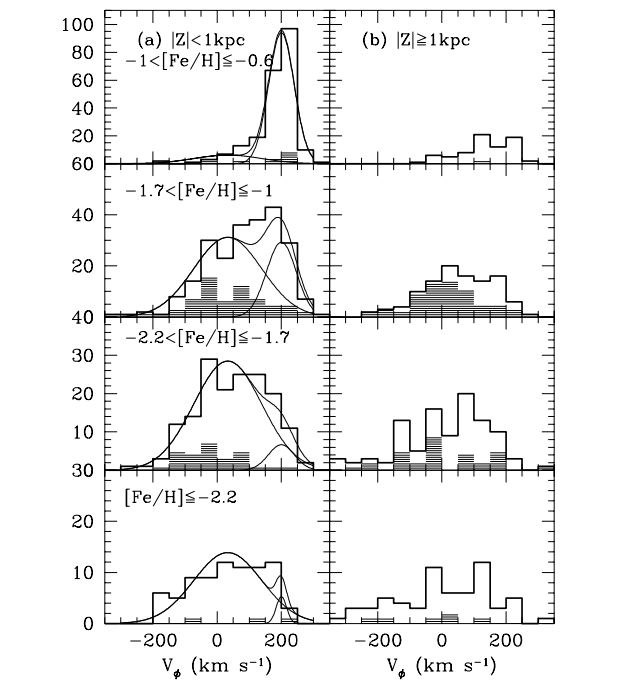Chiba & Beers 2000

### 银晕

#### 元素丰度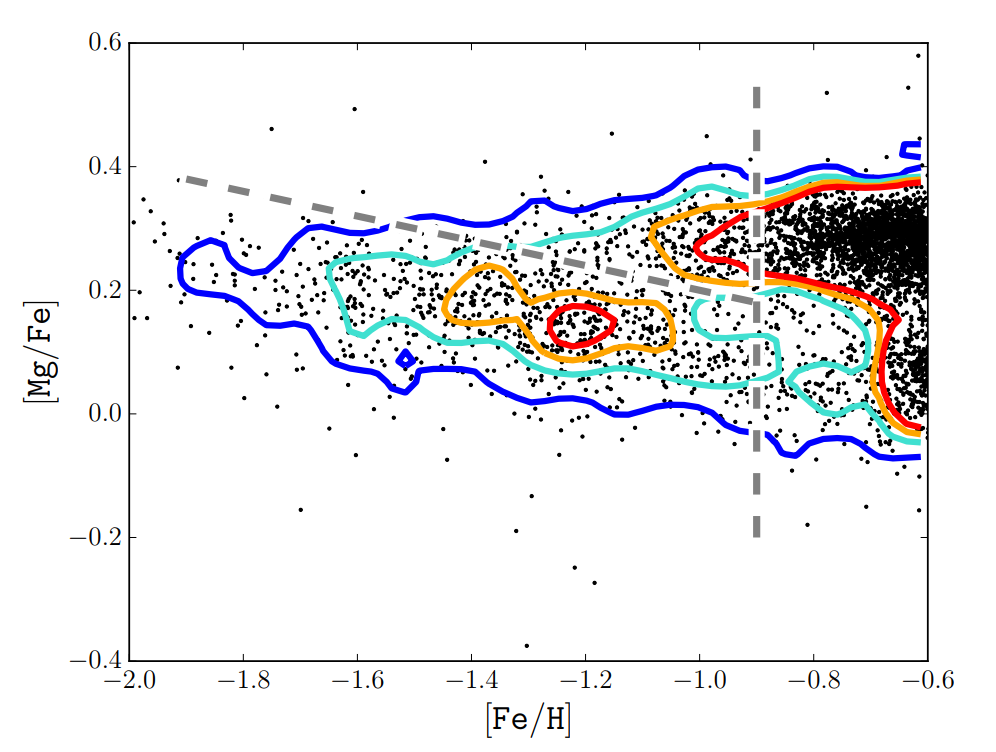Hayes+2017

### 球状星团

#### 水平分支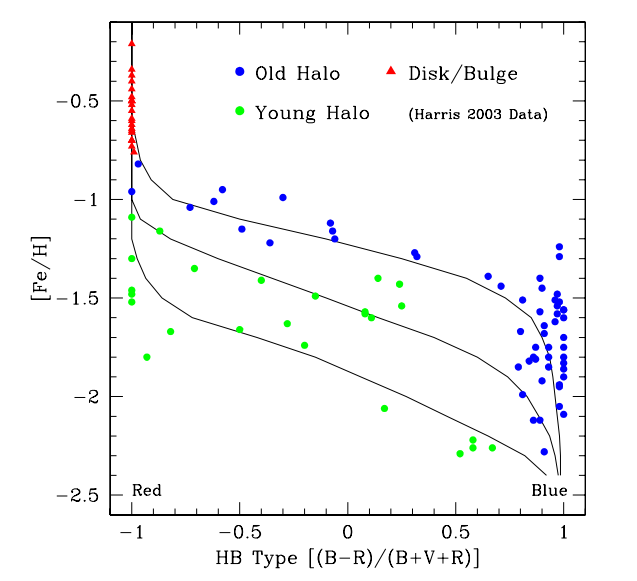Mackey & Gilmore 2004，x轴数值越大越蓝，图中的等年龄线之间最大相差2.2Gyr

#### 多星族性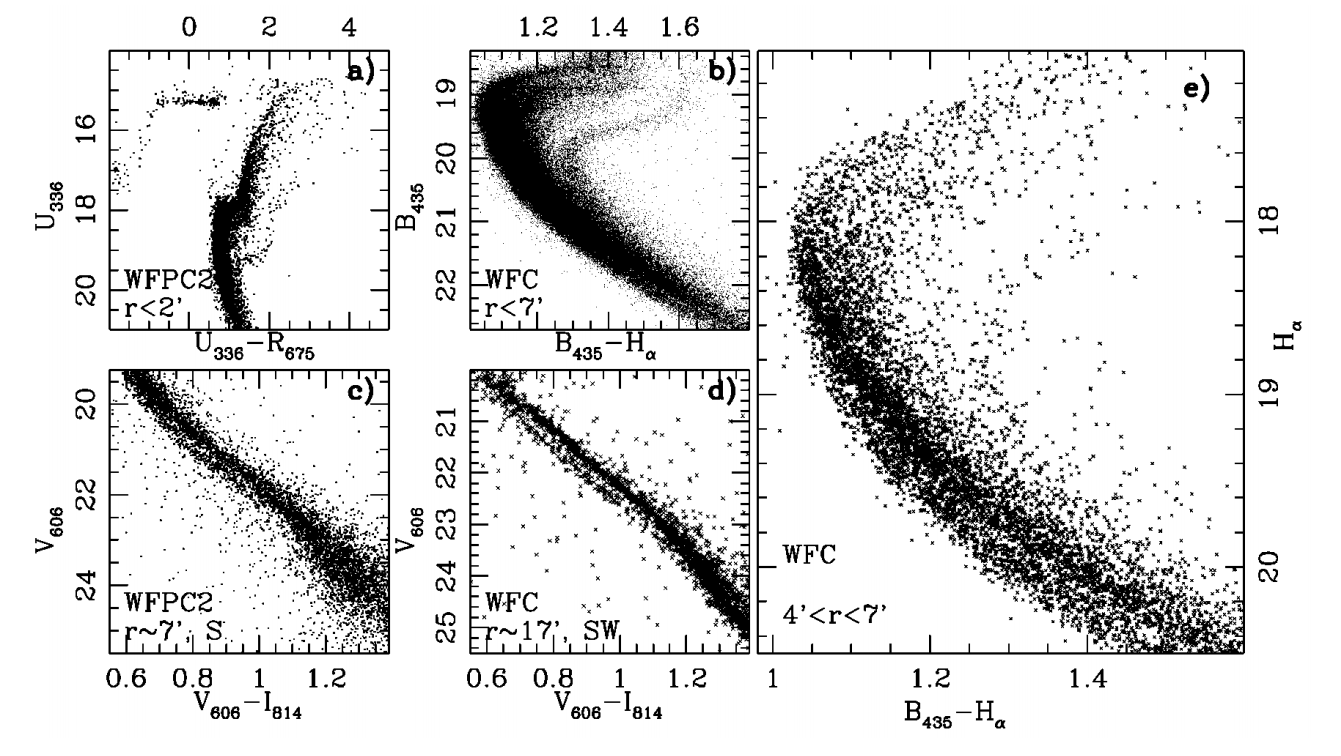Bedin+2004，$$\omega$$ Cen的CMD

### 矮星系

• dE，矮椭圆星系
• dSph，矮椭球星系
• dIrr，矮不规则星系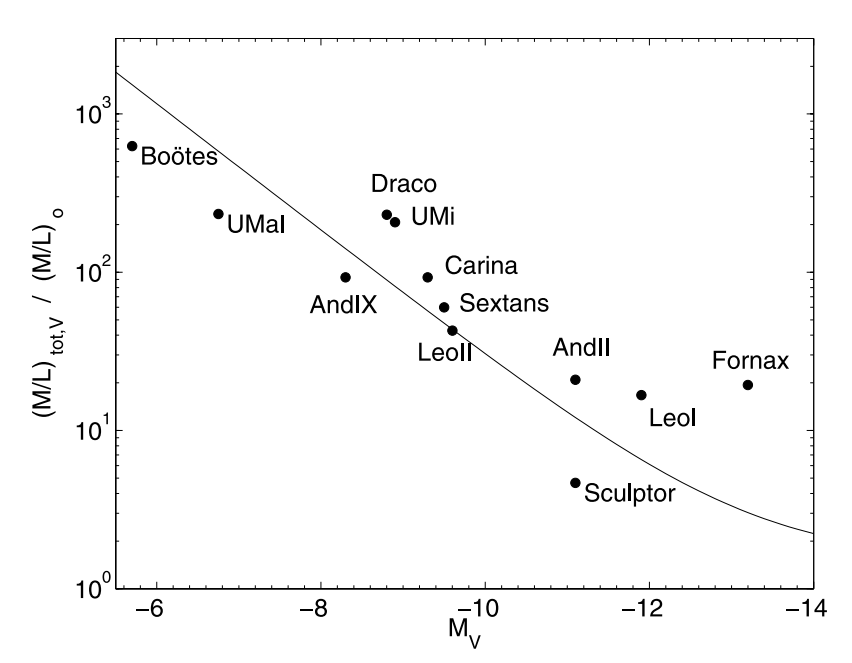Gilmore+2007

## 6 银河系的形成与演化

### 古典理论

#### 快收缩理论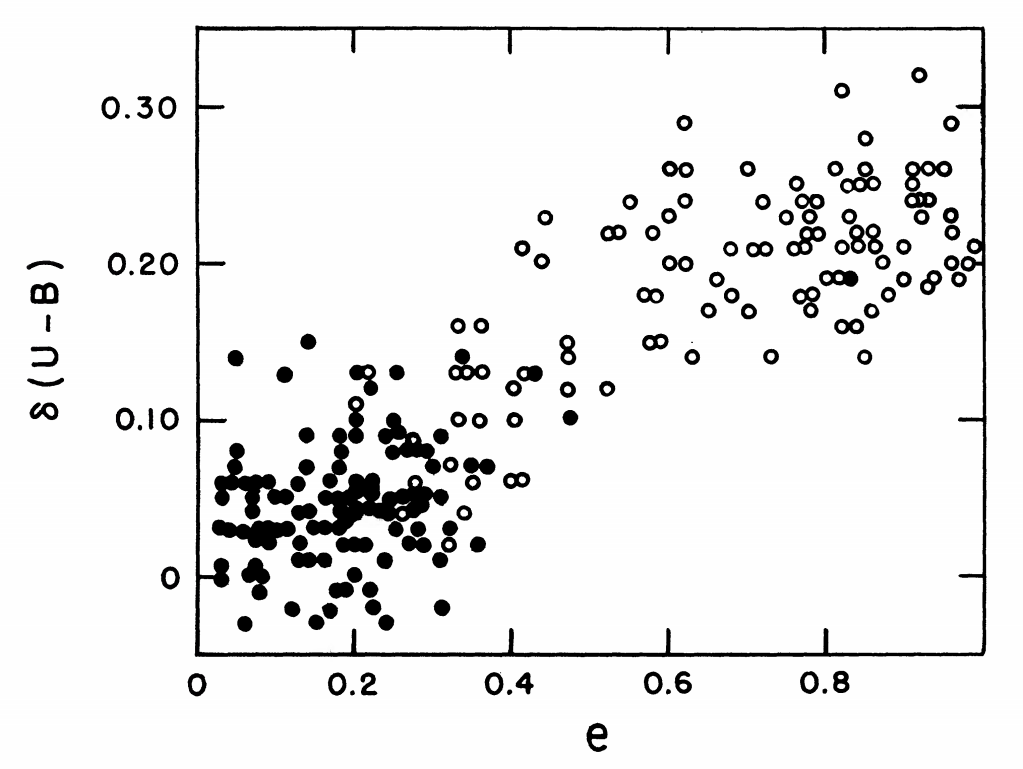Eggen+1962

#### 来，批判一番

ELS理论在后来受到了比较大的质疑。Yoshii & Saio 1979与Norris+1985得出了这样的图：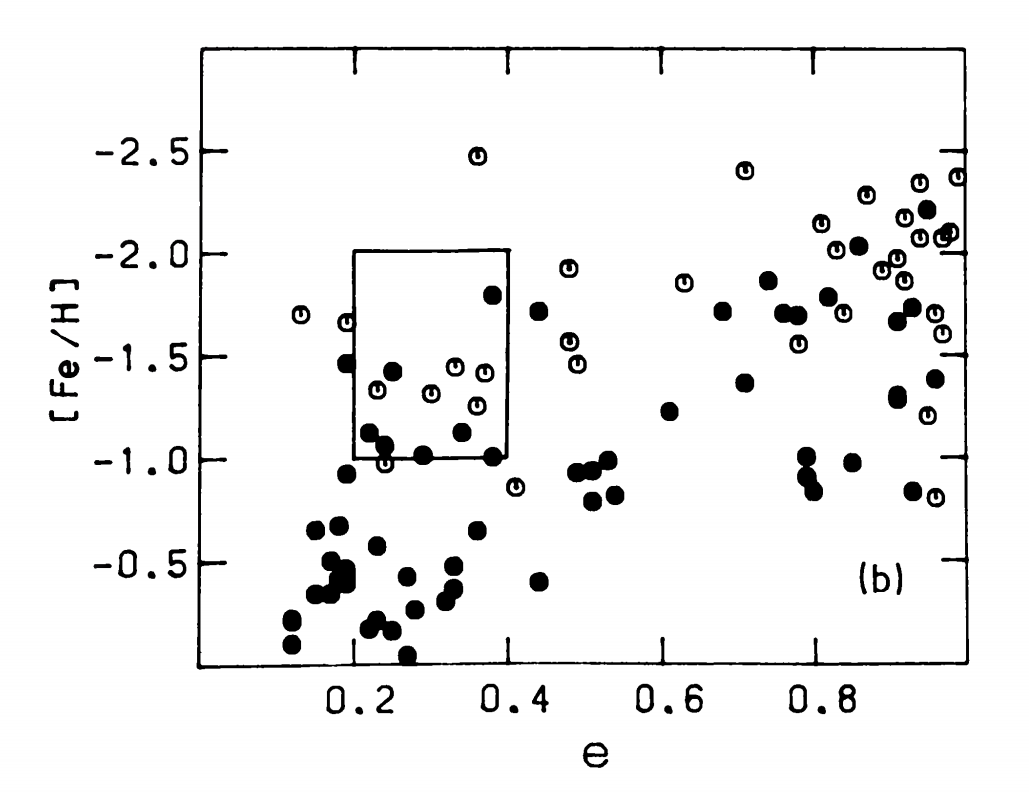Norris+1985

### 现代理论：有关银晕的研究

#### 运动学研究进展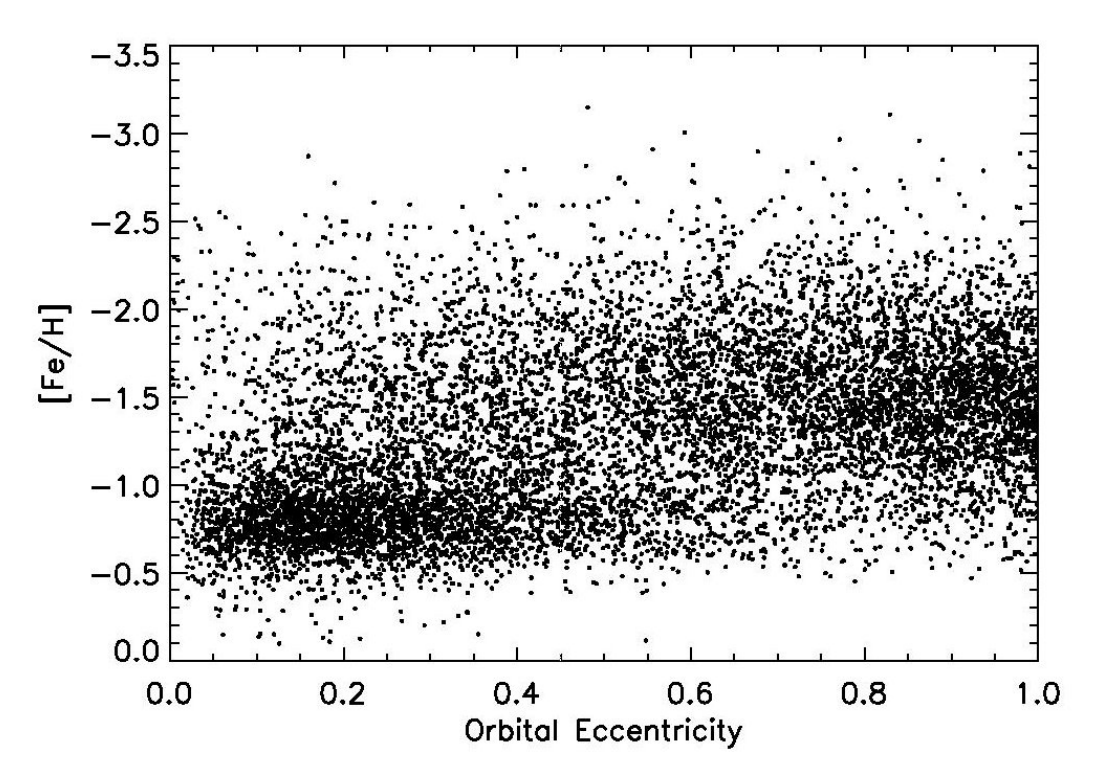Carollo+2007

#### 多部分合并理论的证据

SZ模型被提出之后，人们就开始寻找合并的证据。在有了大规模巡天之后，首先我们可以从恒星的空间分布中找出一些还存在的遗留物。Belokurov+2006就通过SEGUE找到了不少星流，它们就是被并合的矮星系残留的证据。这也从一个侧面证明了合并的力学时标很长，一直到现在。

### 厚盘的形成

• ELS理论的厚盘形成

• SZ理论的厚盘形成

• 下落到银盘的矮星系残骸

• 先有一个盘，被下落到银盘的矮星系残骸之类的加热

• 盘上有分子云clump

### 薄盘的形成

1. $$R$$方向的光度指数下降
2. 平坦的自转曲线
3. 金属丰度约为0，$$\alpha$$比铁比较低
4. $$R$$方向有负的金属丰度梯度

#### 光度的指数下降

1963年Mestel考虑了一个匀质的刚体旋转的气体球，从这开始收缩成一个无限薄的盘，满足了第12条。但是有些细节对不上；在$$R$$方向加上粘性对流可能可以满足。

#### 金属丰度梯度

inside-out的形成。可能是因为内部物质比较丰富，就形成得快一点。

### 遥远星系的观测

• 气体冷却太快，核球太重
• 气体冷却太快，恒星形成太多
• 有cold accretion，形成了纤维状的结构

### 核球的形成

classic bulge就是在银河系形成的时候形成的一个核，而pseudo bulge是bar-盘的相互作用而形成的一个长形的核。现在认为银河系的核主要是pseudo bulge。

# 后面的内容就略过了